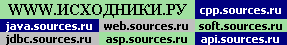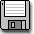Powered byНа Главную Pascal Форум Информер Страны мира
Математика    >>    rmathRider Math Library for TP4+ J. W. Rider 01.12.1990 Три математических модуля: Элементарная математика, Математика Комплексных чисел, Специальные функции. MATH - elementary mathematical routines: Numerical rounding and fractioning; Binary operators; Conditional operators; Polynomial evaluation; Exponential and power-raising functions; Logarithmic functions; Circular trigonometric functions and inverses; Degree/radian conversions for circular trig; Hyperbolic trigonometric functions and inverses. CMATH - complex mathematical routines: Binary operators; Exponenential, power-raising, and logarithmic procedures; Circular and hyperbolic trigonometric procedures and inverses; Conversion functions; Polynomial evaluation. SpecFun - Special functions for mathematical calculations: Discrete combinatorics; Continous "combinatorics"; "Incomplete" versions of gamma; "Incomplete" versions of beta; The "error" function and its complement20k```MATH -- a unit of elementary mathematical routines for Turbo Pascal versions 4, 5, 5.5, and 6. Copyright 1990, by J. W. Rider MATH.PAS provides a library of mathematical constants, type definitions, functions, and procedures for immediate use within contemporary Turbo Pascal programs (tested with versions 5.5 and 6). Routines are provided for the following categories: Numerical rounding and fractioning: ceil, floor, modf, remf, round2, sgn B```